# A Guide to Understand Hooke's Law with Diagram

An object experiences specific changes when there is an application of force to it. These changes are noticeable considering its material. There can be some amount of extension or compression. The equation determining the relation between the force and the extension of the object is known as Hooke's Law. Thus, to learn the properties of materials and the related laws of physics and mechanics, the students must understand Hooke's Law. For a thorough knowledge of this subject, the students can use a Hooke's Law graph.

## 1. The Hooke's Law

In the 19th century, English physicist Robert Hooke established a relationship between the stress and strain of some elements. He put forward two statements called Hooke's Law.

According to him, the force applied to stretch an object is proportionate with the object's extension.

1. As per Hooke's Law first statement, when there is a force applied to elongate or compress a spring, the much-needed force is proportional to the displacement of deformity of the spring.
2. The second statement of Hooke's Law caters to the strain-stress relationship. When an object is within its elastic limit, its strain and stress are in proportion. This property is called elasticity. If someone does not withdraw this stress from the element, the body gets back to its original shape.#### 1.1 The Hooke's Law Experiment

For the Hooke's Law Experiment, the students need to have three springs with two weights of 1N and 2N. At the beginning of the experiment, they can hang the spring without any load. They will not find any expansion there. It stays in a relaxed condition. When there is an application of 1N load on the second spring, the students will notice a certain displacement, and the expansion will be X.

In the third spring, exert a load of 2N. The displacement will be 2X.

It shows that the force exerted on the object is in a proportional relationship with its expansion. When the values are substituted on Hooke's Law, the student will derive the constant.

It is known as the Spring constant of an object. It is dependent on the material of the element. The spring constants of different objects are different.

#### 1.2 The Hooke's Law Equation

Students can understand this equation with the help of two different expressions based on the statements of Hooke's Law.

The equations of Hooke's Law are:

• The first statement of the Hooke's Law suggests, F= -k.x

In this equation, F stands for force; x represents the extension or compression of the spring; k is the spring constant in the equation, denoting the constant of proportionality.

• As per the second statement of Hooke's Law, σ = Eε. In this equation, σ denotes the stress, E represents the modulus of elasticity or Young’s modulus, ε is a dimensionless term here, which stands for the strain.

#### 1.3 The Limitation of Hooke's Law

Though Hooke's Law has wide usage in Engineering and Mechanics, it is not universal. There are certain conditions in which Hooke's Law does not work. Some limitations of Hooke's Law are:

• It is not applicable once the object passes the elastic limit of the material.
• It is accurate when the application on solid objects showing slight deformations.
• On many materials, Hooke's Law is not applicable. They deviate from it even within the elastic limit of their material.
• If an object is stretched beyond its capacity, Hooke's Law does not work on it.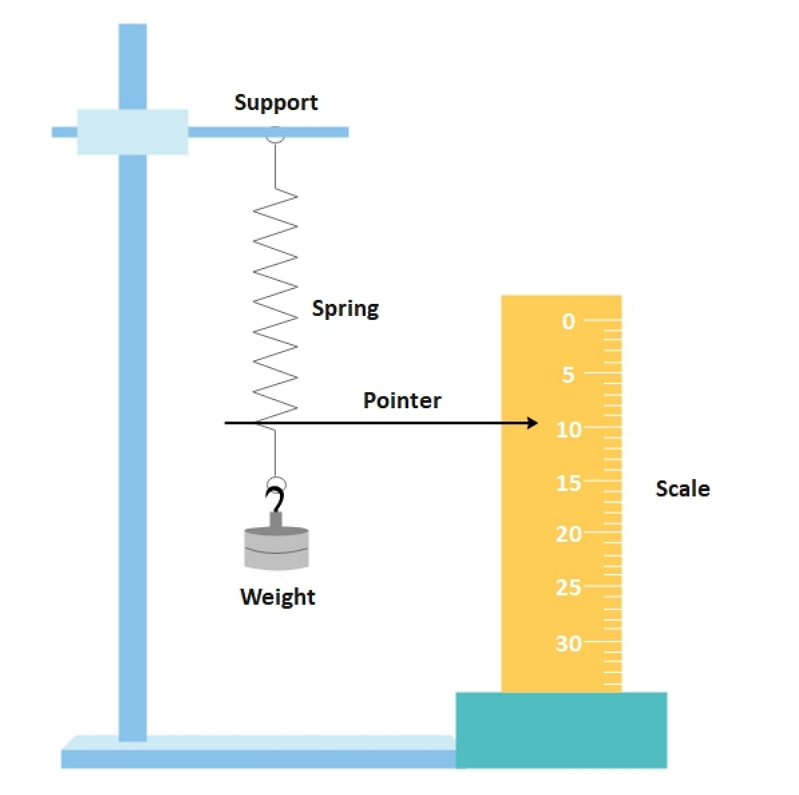Source:EdrawMax Online

## 2. How to Draw the Hooke's Law?

While studying the properties of a material and its strain and stress, the students must learn Hooke's Law. For that, they can use a Hooke's Law graph for their studies. The students can create a Hooke's Law, but the process is challenging.

#### 2.1 How to Create Hooke's Law from Sketch

Here are a few steps the students need to follow to draw a Hooke's Law graph by hand:

Step 1: First, the students need to take a graph paper and then draw the axes with a ruler. They need to draw a vertical line and a horizontal line intersecting each other. The intersecting point is the center (0,0). The vertical line or the Y-axis represents force (N), the horizontal line or X-axis represents the extension (mm) of the material after the force exertion.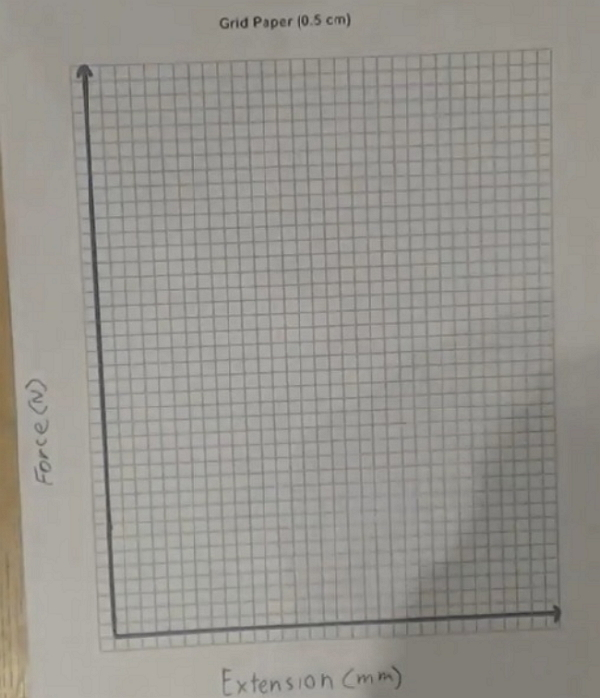Step 2: The students need to do the axis labels after this. They need to mark the places like 0, 5, 10, and so on, on the X-axis. They should leave five units (small boxes of graph paper) gap. Similarly, markings of 0.1, 0.2, 0.3 should be there on the Y-axis of the graph. Students should leave five units’ gap.

Step 3: After the labeling of the axes, the students need to plot the points (5, 0.1), (10, 0.2), (15, 0.3), and so on. Then they need to connect the points, which will form a straight line passing through the center of the graph.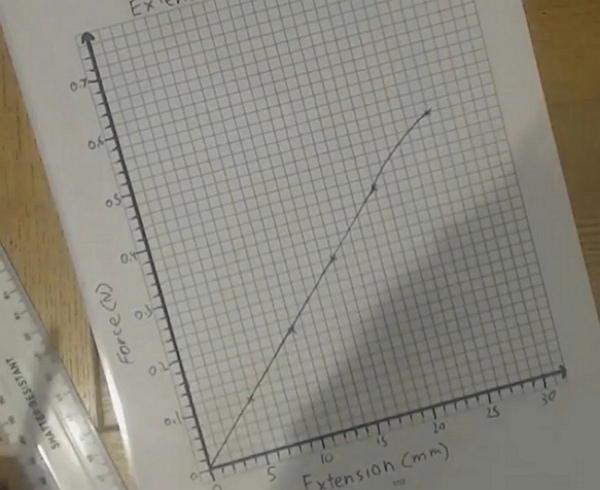Step 4: If the students have the reading from the laboratory, they can also use the values to plot in the graph for Hooke's Law.

#### 2.2 How to Create Hooke's Law Online

Creating a Hooke's Law graph by hand is troublesome, and the students may fail to produce a correct result. To avoid this hassle, they must use the EdrawMax Online tool. It is user-friendly, and the users can comfortably work on it without any prior experience. As one of the most robust diagramming tools available in the market, it has more than 260 different types of diagrams. The tool comes with enormous editable diagrams and templates, beneficial for students, companies, and business owners. It has helped the tool to garner a user base of 24 million. The students can also use this tool while creating their projects. To draw the Hooke's Law graph on the EdrawMax Online tool, the students need to follow a few simple steps:

Step 1: It is pretty smooth to create a Hooke's Law graph on the EdrawMax Online tool. The tool comes with an easy-to-use interface. Thus, it has become a trusted diagramming partner of many companies as well as many individual users. Begin the process by opening the EdrawMax Online tool and then by opening New.Source:EdrawMax Online

Step 2: Under the New option, the students will find the Science and Education tab. The students can go to this tab to find multiple education-related diagrams and graphs which they can use in their studies and projects. To get Hooke's Law graph, the students need to go to the Mechanics option. They can find the Mechanics-related graphs in this part.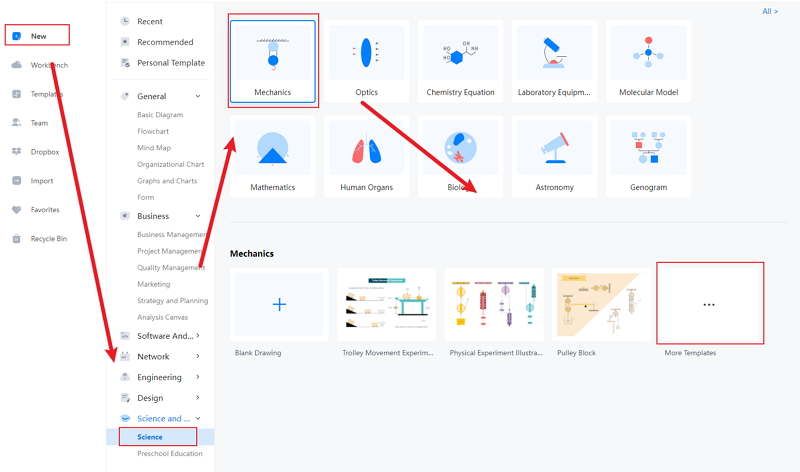Source:EdrawMax Online

Step 3: Now, the students need to select the Hooke's law graph they want. The high-quality Hooke's law graph is perfect to use for projects, dissertation papers, and studies. After choosing the required Hooke's Law graph, they need to modify the image as per their choice.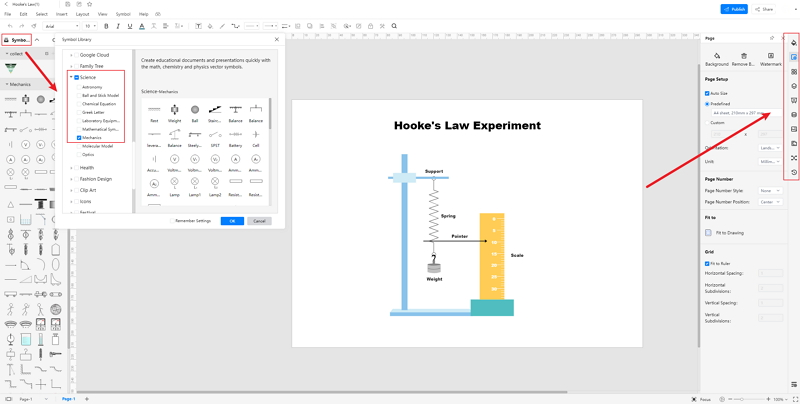Source:EdrawMax Online

Step 4: Once the students complete their diagram, the next and final step is saving it. EdrawMax Online tool allows them to save the file in multiple formats. They can also export the image to use in the future. The tool supports several OS and devices, and hence the students can work anywhere, anytime on this tool. They can also access the diagram from different devices without much hassle.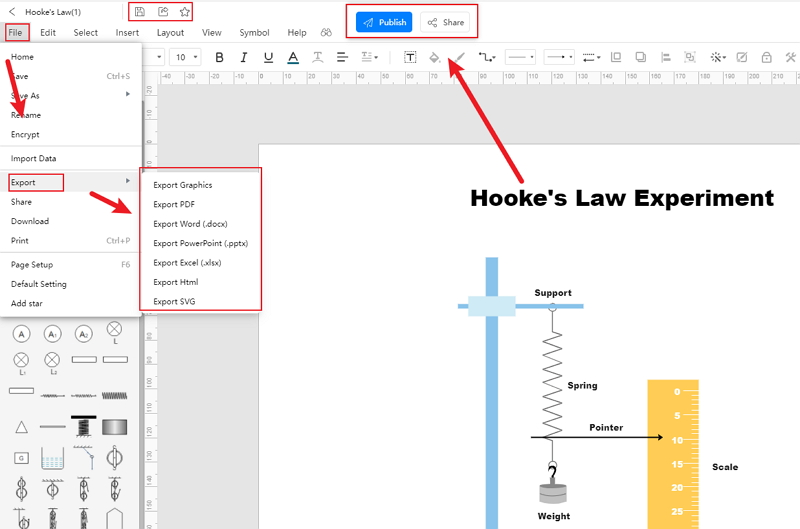Source:EdrawMax Online

## 3. The Application of Hooke's Law

Hooke's Law has a wide range of usage in physics, mechanics, and engineering. The applications of Hooke's Law are:

1. The manometer, spring scale, the balance wheel of a clock is made based on it.
2. It helps in establishing and developing Molecular mechanics.
3. Acoustic and seismology have come from the fundamentals of Hooke's Law.
4. It helps to study physics and explain the nature of an element. It also helps in gathering knowledge regarding the material that helps in its formation.

## 4. Conclusion

For Mechanics, engineering, and Physics students, it is crucial to learn Hooke's Law and how it shows the relationship of the properties of materials. To properly understand the concepts of stress and strain and the property of material-related theories and ideas of mechanics and engineering, the students must study Hooke's Law. To have a better idea about it, the students can use Hooke's Law graph. They can create it by hand, but the students may fail to draw a good one. They need to plot the points aptly to generate a perfect graph. The process is challenging and time-consuming. Thus, the students must use the EdrawMax Online tool. It can help them to get a high-quality Hooke's Law graph without any difficulty.

In conclusion, EdrawMax Online is a quick-start diagramming tool, which is easier to make Hooke's Law diagram and any 280 types of diagrams. Also, it contains substantial built-in templates that you can use for free, or share your science diagrams with others in our template community.

### Related Articles